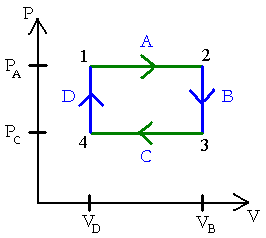Explore BrainMass

# PV Diagrams

A pressure volume diagram is used to describe corresponding changes in volume and pressure in a system. They are commonly used in thermodynamics, cardiovascular physiology and respiratory physiology.

PV diagrams were originally called indicator diagrams. They were developed in the 18th century as tools for understanding the efficiency of steam engines. It was developed by James Watt and John Southern.

PV diagrams can be used to estimate the net work performed by a thermodynamic cycle. The net work is the area enclosed by the PV curve in the diagram. This usage derived from the development of indicator diagrams which were used to estimate the performance of a steam engine. The diagram records the pressure of steam versus the volume of steam in a cylinder throughout a piston’s cycle of motion in a steam engine.

On a pressure volume diagram, the volume is on the x-axis and the pressure is on the y-axis.### calcuation of heat and work in the piston-cylinder problem

Please show all the work. A piston-cylinder device whose piston is resting on a set of stops initially contains 3 kg of air at 2 bar and 27 degree Centigrades. The mass of the piston is such that a pressure of 4 bar is required to move it. Heat is now transferred to the air until the volume doubles. Determine the work done by

### Thermodynamic process

See attached diagram. 1. The figure shows a thermodynamic process followed by 150mg of helium. a) Determine the pressure (in atm) of the gas at points 1, 2, and 3. p1,p2,p3=________atm b) Determine the temperature (in celsius) of the gas at points 1, 2, and 3. T1,T2,T3=________ celsius c) Determine the volume

### Thermodynamics Example Problems

1 mole of a perfect gas for which Cv = 3R/2, independent of temp., is taken from a temp of 100 K and pressure of 10^5 Pa to 400 K and 8*10^5 Pa by two different paths. 1. It goes at constant volume from 100 K to 400 K and then isothermally to the final pressure. 2. It goes at constant pressure from 100 K to 400 K an

### PV-Diagram and Ideal Gas

Four moles of O2 are taken from A to B in the process shown in the PV-diagram below. Assume the gas may be treated as ideal. a) What is the heat flow during this process (include the sign)? b) Does heat enter or leave the gas?

### Ideal Gas Model, Quasi-Static Processes

The Bermuda Triangle (see attachment) A cylinder with initial volume V contains a sample of gas at pressure p. The gas is heated in such a way that its pressure is directly proportional to its volume. After the gas reaches the volume 3V and pressure 3p, it is cooled isobarically to its original volume V. The gas is then coole

### Ideal Gas Model Question, Quasi-Static Question

The Bermuda Triangle (see attachment) A cylinder with initial volume V contains a sample of gas at pressure p. The gas is heated in such a way that its pressure is directly proportional to its volume. After the gas reaches the volume 3V and pressure 3p, it is cooled isobarically to its original volume V. The gas is then coole